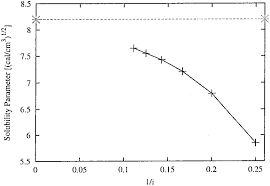## How to Calculate and Solve for Standing Gas Solubility Parameter | Nickzom CalculatorThe image above represents the standing gas solubility parameter.

To compute for the standing gas solubility parameter, two essential parameters are needed and these parameters are API Gravity (°API) and Temperature (°Rankine) (T).

The formula for calculating the standing gas solubility parameter:

x = 0.0125°API – 0.00091(T – 460)

Where:

x = Standing Gas Solubility Parameter
°API = API Gravity
T = Temperature (°Rankine)

Let’s solve an example;
Given that the API Gravity is 22 and the temperature is 120.
Find the standing gas solubility parameter?

This implies that;

°API = API Gravity = 22
T = Temperature (°Rankine) = 120

x = 0.0125°API – 0.00091(T – 460)
x = 0.0125(22) – 0.00091(120 – 460)
x = 0.275 – 0.00091(-340)
x = 0.275 – -0.3094
x = 0.5844

Therefore, the standing gas solubility parameter is 0.5844.